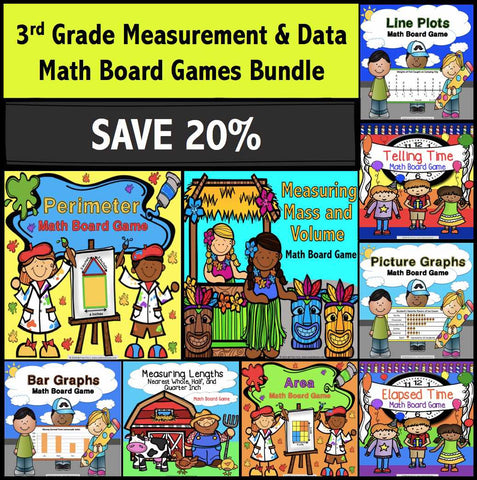## 3rd Grade Measurement and Data Math Games Bundle

• \$2400
• Save \$6.25

3rd Grade Measurement and Data Math Games Bundle gives students a fun way to practice all of the 3rd grade Common Core Measurement and Data standards. This bundle contains nine measurement and data board games.

Each game contains at least 30 word problem game cards and a game board to help your students practice that particular skill. These measurement and data games are perfect to use in small groups, as center activities, or as informal assessment tools.

Plus, you'll get 20% off the individual prices of these math board games when you buy this bundle!

Want to learn more about what you'll get? Click on the links below to view the individual previews for each board game. Remember, you'll get all 9 board games when you purchase the bundle.

Common Core 3.MD.A.1 - Tell and write time to the nearest minute and measure time intervals in minutes. Solve word problems involving addition and subtraction of time intervals in minutes.
Telling Time Board Game
Elapsed Time Board Game

Common Core 3.MD.A.2 - Measure and estimate liquid volumes and masses of objects using standard units of grams (g), kilograms (kg), and liters (l).1 Add, subtract, multiply, or divide to solve one-step word problems involving masses or volumes that are given in the same units.
Measuring Mass and Volume Board Game

Common Core 3.MD.B.3 - Draw a scaled picture graph and a scaled bar graph to represent a data set with several categories. Solve one- and two-step "how many more" and "how many less" problems using information presented in scaled bar graphs.
Picture Graphs Board Game
Bar Graphs Board Game

Common Core 3.MD.B.4 - Generate measurement data by measuring lengths using rulers marked with halves and fourths of an inch. Show the data by making a line plot, where the horizontal scale is marked off in appropriate units— whole numbers, halves, or quarters.
Measuring Lengths Board Game
Line Plots Board Game

Common Core 3.MD.C.5 - Recognize area as an attribute of plane figures and understand concepts of area measurement.
Common Core 3.MD.C.6 - Measure areas by counting unit squares (square cm, square m, square in, square ft, and improvised units).
Common Core 3.MD.C.7 - Relate area to the operations of multiplication and addition.
Area Board Game

Common Core 3.MD.D.8 - Solve real world and mathematical problems involving perimeters of polygons, including finding the perimeter given the side lengths, finding an unknown side length, and exhibiting rectangles with the same perimeter and different areas or with the same area and different perimeters.
Perimeter Board Game